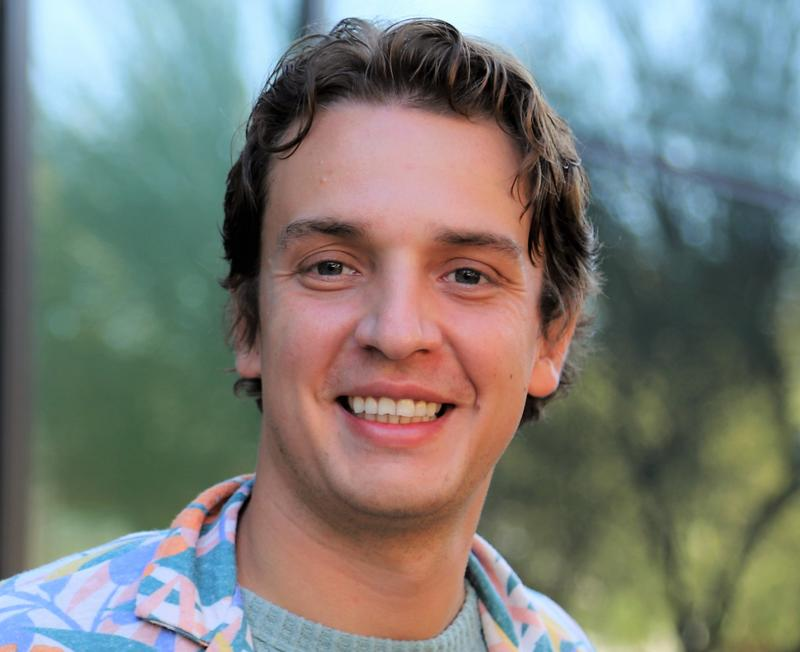# Nikola Kovachki### Abstract

In the first part of this talk, we consider the problem of learning general non-linear operators acting between two infinite-dimensional spaces of functions defined on bounded domains. We generalize the notation of a neural network from a map acting between Euclidean spaces to one acting between function spaces. The resulting architecture has the property that it can act on any arbitrary discretization of a function and produce a consistent answer. We show that the popular transformer architecture is a special case of our model once discretized. We prove universal approximation theorems for our network when the input and outputs spaces are Lebesgue, Sobolev, or continuously differentiable functions of any order. Furthermore, we show dimension-independent rates of approximation, breaking the curse of dimensionality, for operators arising from Darcy flow, the Euler equations, and the Navier-Stokes equations. Numerical experiments show state-of-the-art results for various PDE problems and successful applications in down-stream tasks such as multi-scale modeling and solving inverse problems. In the second part of this talk, we consider the problem of sampling a conditional distribution given data from the joint using a transport map. We show that a block-triangular structure in the transport map is sufficient for this task, even in infinite-dimensions, and prove posterior-consistency results for measures with unbounded support. We obtain explicit rates of approximation when the transport map is parameterized by a ReLU network. Numerically, we successfully apply our approach to uncertainty quantification for supervised learning and Bayesian inverse problems arising in imaging and PDEs.

### Our speaker

Nikola a final-year PhD student in applied mathematics at Caltech working on machine learning methods for the physical sciences in theory and practice.

To become a member of the Rough Path Interest Group, register here for free.